NumberSpiral.com
 Home Download Contact Links Next page

CONTENTS4.  Factors on product curves

 On this page we'll figure out why numbers on product curves have the factors that they do. The clue to the mystery is found in the first curves we looked at, the product curves on the square side.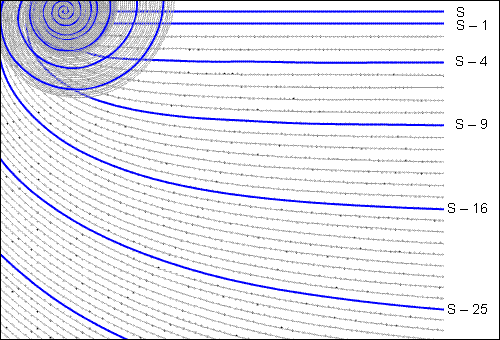Figure 1

 The clue is the offsets. All of them are perfect squares. Since the numbers on the top line (curve S) are also perfect squares, every number on these product curves is equal to the difference between two squares. Such numbers can always be factored.VortexTo draw these curves with Vortex, enter "s" and "s-–4" in the command window. The black arrow will not appear.
Figure 2

 Let's take 117 as an example. Since it's located on curve S – 4, we know it's four less than the perfect square on the top line, which happens to be 121. Writing these facts in the form of an equation, we get: 117 = 121 – 4 Since 121 and 4 are perfect squares, we can rewrite the equation like this: 117 = 112  –  22 Whenever you have one square minus another square, you can factor like so: 117 = (11 + 2) x (11 – 2) And therefore we conclude that: 117 = 13 x 9 And that's the story. Every integer on the square-side product curves can be factored this way. But that's not the whole story. This method applies to all product curves, including those on the pronic side. At first glance it's difficult to see how this can be true. Take a look: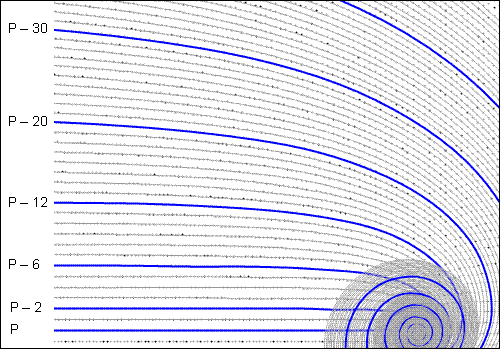VortexTo draw these curves with Vortex, enter "off 1/2" in the command window.
Figure 3

 The offsets on the pronic side are 2, 6, 12, 20, 30, etc. Needless to say, those numbers aren't perfect squares. But those offsets are measured from curve P. Those offsets don't matter right now. The ones that matter are measured from angle 1/2 (the angle that these curves are offset from). We didn't need to think about this distinction with curve S because curve S sits on top of its angle. But curve P is offset from its angle, like so: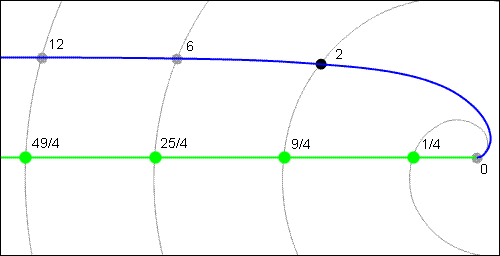VortexTo draw these curves with Vortex, enter "ang 1/2" and "p" in the command window. (You won't see numbers on the green line — I drew them for this illustration with Photoshop.)
Figure 4

 I've drawn numbers on the angle (shown in green) so we can measure the offsets between the angle and its curves. How do I know these numbers? Because back on page one, when I decided how the spiral is constructed, I said that every number is placed on the angle which equals its square root. (I said it in the form of an equation down in the details box.) This means that to find the number on any given angle, all we have to do is square the angle. Of course this is true only if we measure angles in rotations. And that is why I measure angles in rotations on this website, in case you've been wondering. Let's take the first green dot (moving from right to left) as an example. At that location, the spiral (the gray coil) has made exactly one half of a rotation, so the angle is 1/2. One half squared is one quarter, so the number on that dot is one quarter. At the next green dot the spiral has made one and a half rotations, so the total angle (not merely its fractional part) at that location is 3/2. If we square that, we get 9/4. That's the number on the second dot. Using the same reasoning for the other dots, the whole sequence goes like this as we travel outward on angle 1/2: (1/2)2 = 1/4 (3/2)2 = 9/4 (5/2)2 = 25/4 (7/2)2 = 49/4 (9/2)2 = 81/4 (11/2)2 = 121/4 etc. This is a fairly regular pattern, but if you you hold nature to the same high standards as I do, you may find it a bit worrying that all the numerators are odd. Where are the even ones? Have no fear; they will turn up before the end of this page. Now we can calculate the real offsets. For the first non-zero pronic, 1x2 = 2, the offset is 9/4 – 2  =  9/4 – 8/4  =  1/4 Now that we know that the real offset is 1/4, we can express 2 as the difference between two squares: 2  =  9/4 – 1/4  = (3/2)2 – (1/2)2  = (3/2 + 1/2) x (3/2 - 1/2)  = 2 x 1 Except for the fact that we're working with fractions, this is exactly what we did with 117 on curve S – 4. We're almost finished with this topic, but not quite. We still need to round up the missing positive numerators of the pronics.

 To find them we must consider the angle 1/2 as part of a set. There are two angles with denominator 2 on the spiral — 0/2 and 1/2 — and we have to analyze them simultaneously. This may seem a bit silly since angle 0/2 is really angle zero, which we already examined at the top of this page — it's good old curve S. But if we think of it as angle 0/2, the arithmetic works out with different numbers, and we become aware that the pattern is utterly complete and orderly. In addition to the aesthetics, there is also a practical reason for looking at all the angles with a given denominator as a single group; we will need to do this a little later in order to predict the locations of offset curves from the coefficients of their quadratic formulas. The following table shows the beginning of the complete system of offset curves for angles with denominator 2. Note that all the offsets, whether they belong to curves on the pronic or square side, consist of fractions with a perfect square in the numerator. It is this perfect square in the numerator of the offset which distinguishes a purely composite offset curve from ordinary ones. Also note that every possible square is included. Squares of even numbers are on the square side, and squares of odd numbers are on the pronic side. The sytem is completely regular.Angle Number on angle Offset 0/4 (curve S) Offset 1/4 (curve P) Offset 4/4 (curve S–1) Offset 9/4 (curve P–2) Offset 16/4 (curve S–4) Offset 25/4 (curve P–6) Offset 36/4 (curve S–9) 0 0 0x0 1/2 1/4 0x1 2/2 4/4 = 1 1x1 0x2 3/2 9/4 1x2 0x3 4/2 16/4 = 4 2x2 1x3 0x4 5/2 25/4 2x3 1x4 0x5 6/2 36/4 = 9 3x3 2x4 1x5 0x6 7/2 49/4 3x4 2x5 1x6 8/2 64/4 = 16 4x4 3x5 2x6 1x7 9/2 81/4 4x5 3x6 2x7 10/2 100/4 = 25 5x5 4x6 3x7 2x8 11/2 121/4 5x6 4x7 3x8 12/2 144/4 = 36 6x6 5x7 4x8 3x9 13/2 169/4 6x7 5x8 4x9To make that table clearer, here is the same information in the form of equations: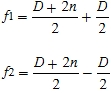...where D is the difference between factors and n is an integer that varies from zero to infinity. Here is another function for the same purpose that plots the factors directly from any real number x on the spiral: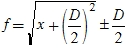1  2  3  4  5  6  7  8  9  10   NextCopyright © 2003, 2007 Robert Sacks